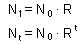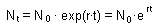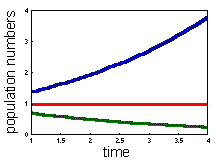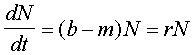## Exponential Model

Exponential model is associated with the name of Thomas Robert Malthus (1766-1834) who first realized that any species can potentially increase in numbers according to a geometric series. For example, if a species has non-overlapping populations (e.g., annual plants), and each organism produces R offspring, then, population numbers N in generations t=0,1,2,... is equal to:When t is large, then this equation can be approximated by an exponential function:There are 3 possible model outcomes:1. Population exponentially declines (r < 0)
2. Population exponentially increases (r > 0)
3. Population does not change (r = 0)
Parameter r is called:
• Malthusian parameter
• Intrinsic rate of increase
• Instantaneous rate of natural increase
• Population growth rate
"Instantaneous rate of natural increase" and "Population growth rate" are generic terms because they do not imply any relationship to population density. It is better to use the term "Intrinsic rate of increase" for parameter r in the logistic model rather than in the exponential model because in the logistic model, r equals to the population growth rate at very low density (no environmental resistance).

Assumptions of Exponential Model:

1. Continuous reproduction (e.g., no seasonality)
2. All organisms are identical (e.g., no age structure)
3. Environment is constant in space and time (e.g., resources are unlimited)
However, exponential model is robust; it gives reasonable precision even if these conditions do not met. Organisms may differ in their age, survival, and mortality. But the population consists of a large number of organisms, and thus their birth and death rates are averaged.

Parameter r in the exponential model can be interpreted as a difference between the birth (reproduction) rate and the death rate:where b is the birth rate and m is the death rate. Birth rate is the number of offspring organisms produced per one existing organism in the population per unit time. Death rate is the probability of dying per one organism. The rate of population growth (r) is equal to birth rate (b) minus death rate (m).

Applications of the exponential model

• microbiology (growth of bacteria),
• conservation biology (restoration of disturbed populations),
• insect rearing (prediction of yield),
• plant or insect quarantine (population growth of introduced species),
• fishery (prediction of fish dynamics).

Alexei Sharov 2/03/97